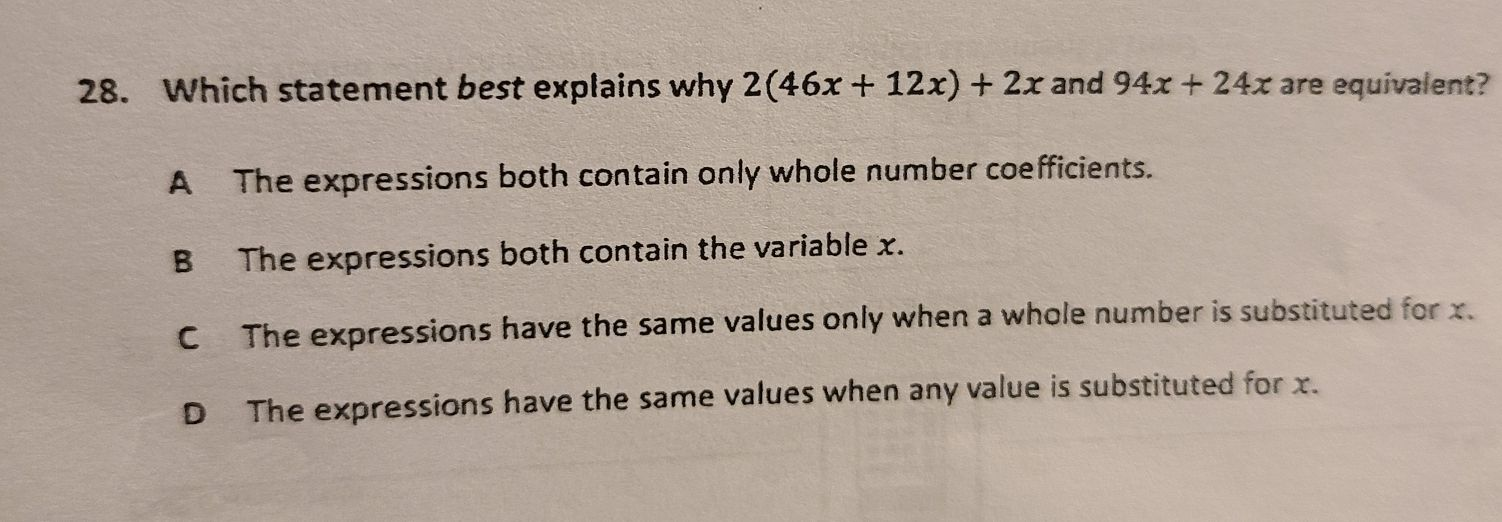### ¿Todavía tienes preguntas de matemáticas?

Pregunte a nuestros tutores expertos
Algebra
Pregunta28. Which statement best explains why $$2 ( 46 x + 12 x ) + 2 x$$ and $$94 x + 24 x$$ are equivalent?

A The expressions both contain only whole number coefficients.

B The expressions both contain the variable $$x$$ .

C The expressions have the same values only when a whole number is substituted for $$x$$ .

D The expressions have the same values when any value is substituted for $$x$$ .